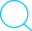22 Apr 2018

# Making use of Pytorch Distribution's hidden gem - validate_args

This post is aimed at helping someone who runs into some misleading `RuntimeError`s when using PyTorch’s `torch.distributions` functions. Specifically, one particular trap/mis-leading- error-message with `torch.distributions.MultivariateNormal` is discussed in this post and may apply to other distributions as well.

## Problem description

Calling `torch.distributions.MultivariateNormal.log_prob(numpy_array)` produces `RuntimeError: Can't call numpy() on Variable that requires grad. Use var.detach().numpy() instead` if the parameter(s) require gradients.

## Code example

``````import torch
sigma = torch.eye(2)
distrib = torch.distributions.MultivariateNormal(mu, sigma)
a = distrib.sample()
#loss = - distrib.log_prob(a)  # This will run fine because a is a Tensor
loss_np = - distrib.log_prob(a.numpy())
``````

Produces `RuntimeError: Can't call numpy() on Variable that requires grad. Use var.detach().numpy() instead.` with the following Traceback:

``````Traceback (most recent call last):
File "distrib_debug.py", line 6, in <module>
loss_np = - distrib.log_prob(a.numpy())
File "~/python3.5/site-packages/torch/distributions/multivariate_normal.py", line 181, in log_prob
diff = value - self.loc
File "~/python3.5/site-packages/torch/tensor.py", line 376, in __array__
return self.cpu().numpy()
RuntimeError: Can't call numpy() on Variable that requires grad. Use var.detach().numpy() instead.
``````

Here, we are interested in learning the parameters `mu` and so we set the `requires_grad = True` in order to get the gradients computed. But, when we call `distrib.log_prob(a.numpy())`, we get the above `RuntimeError` which is misleading since the `RuntimeError` is suggesting us to `detach()` the `mu` Tensor (Variable) from the computation graph, convert it to numpy array and then call `log_prob(...)` which means the gradients for `mu` will not be computed – which is not what we want.

This will work fine if `mu` does not require gradients. For example, the following code will run fine:

``````import torch
mu = torch.tensor([0.0, 0.0])  # Note: requires_grad is False by default
sigma = torch.eye(2)
distrib = torch.distributions.MultivariateNormal(mu, sigma )
a = distrib.sample()
loss_np = - distrib.log_prob(a.numpy())
``````

We will get the above mis-leading `RuntimeError` if even one of the parameters (`mu` or `sigma`) requires gradient to be computed.

You may notice from the comment in the first code snippet in this post that calling `distrib.log_prob(a)` where `a` is a Tensor will not cause this error. But this is not intuitive or explained in the logs even though we can use the `validate_args` parameter to explictly require validating the input arguments to the distribution functions. The useful`validate_args` argument was introduced in this PR and was merged in March 2018.

In summary, to avoid some head aches due to mis-leading `RuntimeError` messages, you can set the `validate_args` argument to `True` when initializing a distribution like this:

`distrib = torch.distributions.MultivariateNormal(mu, sigma, validate_args=True)`

This will perform several sanity checks on the supplied arguments including boundary conditions and will raise useful/sensible value exceptions before they turn into puzzling `RuntimeErrors`. I think by default the validation of the input arguments to the distribution functions are disabled due to performance overhead. Good choice but stating this clearly in the documentation will help the users I guess.